Articles

# Quantifying seasonal and diurnal variation of stomatal behavior in a hydraulic-based stomatal optimization model

## Abstract

Plant responses to drought occur across many time-scales, with stomatal closure typically considered to be a critical short-term response. Recent theories of optimal stomatal conductance linked to plant hydraulic transport have shown promise, but it is not known if stomata update their hydraulic “shadow price” of water use (marginal increase in carbon cost with a marginal drop in water potential) over days, seasons, or in response to recent drought. Here, I estimate the hydraulic shadow price in five species – two semi-arid gymnosperms, one temperate and two tropical angiosperms – at daily timescales and in wet and dry periods. I tested whether the shadow prices varies predictably as a function of current and/or lagged drought conditions. Diurnal estimates of the hydraulic shadow price estimated from observed stomatal conductance, while variable, did not vary predictably with environmental variables. Seasonal variation in shadow price was observed in the gymnosperm species, but not the angiosperm species, and did not meaningfully influence prediction accuracy of stomatal conductance. The lack of systematic variation in shadow price and high predictive ability of stomatal conductance when using a single set of parameters further emphasizes the potential of hydraulic-based stomatal optimization theories.

## Introduction

Stomatal response to environmental variation has a major influence on ecosystem carbon, water, and energy fluxes, and thus is important for global carbon and water cycles (Berry et al., 2010). Human-caused climate change is expected to intensify the hydrological cycle, which will greatly affect ecosystems in the 21st century (Field et al., 2014). Plant stomatal conductance is a central physiological process that will mediate plant responses to changes in climate mean and extremes (Farquhar & Sharkey, 1982; Field et al., 1995; Franks et al., 2013; Keenan et al., 2013). Thus, stomatal behavior is likely to influence ecosystem response to climate change and also carbon cycle feedbacks of the terrestrial biosphere (Berry et al., 2010; Franks et al., 2013; Swann et al., 2016).

Stomatal response to drought – some combination of increasing atmospheric water demand through vapor pressure deficit (VPD) or decreasing soil water availability through falling soil water potential (ψs) – is a key component of plant drought responses (Tuzet et al., 2003; Sperry et al., 2016; Martin-StPaul et al., 2017). Stomatal closure is a critical way in which plants curtail water loss to avoid damage at shorter time-scales of seconds to days (Martin-StPaul et al., 2017). However, stomatal responses occur alongside a suite of longer time-scale responses, including canopy area adjustment (e.g. leaf shedding) (Wolfe et al., 2016), carbon allocation to increase root water uptake (Ledo et al., 2018), osmotic adjustment to tolerate lower water potentials (Bartlett et al., 2014), gene regulation and hormonal responses (Xu et al., 2010; Brodribb et al., 2014, 2016), and plastic changes in xylem anatomy over multiple years (Corcuera et al., 2004; Anderegg, 2015). Because stomatal conductance is influenced by a suite of whole-plant traits and signals, for example leaf water potential that is mediated by hydraulic transport from roots to leaves (e.g. Sperry et al., 2016), longer-term changes in plant drought responses have the potential to alter stomatal sensitivity to the environment. Thus, stomata have the opportunity to behave in a “Bayesian” manner by updating their behavior (e.g. water potentials at which stomatal closure begins) based on previous environmental conditions the plant has experienced, likely mediated by these longer-term plant responses such as biosynthesis of the hormone ABA

The vast majority of ecosystem models use empirical algorithms of stomatal conductance (Ball et al., 1987; Leuning, 1995; Sellers et al., 1996). Such models show reasonable predictive skill over the training conditions but may not be appropriate or skillful in prediction of stomatal conductance in changing and novel environmental conditions. In particular, many empirical models do not capture drought responses with high fidelity, likely due to a lack of representation of soil water potential and its impact on leaf water potential (Anderegg et al., 2017). Furthermore, it is not well-known how often or in what conditions the parameters of empirical models – for example, the slope of stomatal response to relative humidity or vapor pressure deficit – might vary, mediated by some of the well-documented processes described above, and thus would need to be “updated” in an ecosystem model to maintain predictive ability.

Optimal stomatal theories show promise for predicting stomatal conductance in future climates based on linking physiological processes with an evolutionary optimization to maximize plant fitness in varying environments (Medlyn et al., 2011; Prentice et al., 2014; Buckley et al., 2017; Anderegg et al., 2018; but see . A recent “carbon maximization” (CM) optimization has been proposed (Wolf et al., 2016) that posits that plants maximize carbon gain (AN) minus the risk of hydraulic damage driven by low plant water potential (Θ(ψ)). This “risk of hydraulic damage” term may encompass a number of potential physiological mechanisms and is expected to increase sharply as water potentials decline. It is likely an integrated risk term, and thus is not tied to the probability or damage of a single event/outcome, but instead captures a suite of carbon costs that are likely to occur as hydraulic damage progresses. These may include the carbon costs of refilling embolized xylem (Broderson and McElrone, 2013), rebuilding new xylem to restore water transport after embolism (Brodribb and Cochard, 2009), direct damage of photosynthesis by low water potentials (Flexas et al., 2002), impaired phloem transport at low water potentials (Huang et al., 2018), and increased risk of drought-induced mortality at low water potentials (Anderegg et al., 2016; Martin-StPaul et al., 2017), among others.

This CM optimization (also called the “gain-risk” algorithm in Sperry et al. 2017) shows similar responses to environment as classic empirical models (Wolf et al., 2016; Sperry et al., 2017), reproduced hydraulic and gas exchange responses to a controlled drought experiment in Populus tremuloides with high accuracy (Venturas et al., 2018), and provided a major improvement in predictive ability (increase in R2 of 0.11-0.25) over the classic marginal water use efficiency optimization when tested against a dataset of 34 woody plant species spanning global biomes (Anderegg et al., 2018). Because plant hydraulic algorithms are increasingly being incorporated into ecosystem and land surface models (Bonan et al., 2014; Xu et al., 2016; Fisher et al., 2018), this CM optimization has strong promise to link key components of plant drought physiology such as hydraulic traits and differences across species with gas exchange in a consistent framework that improves model prediction in novel climates (Anderegg et al., 2018).

Several key unresolved questions remain, however, concerning the CM optimization at longer time-scales and in varying environments. In particular, do these optimal stomatal parameters vary either 1) directly in response to drought drivers (i.e. VPD and/or ψs) and/or 2) in lagged response to drought drivers? This allows the examination of an interesting question: are stomata Bayesian in that they update their hydraulic risk “shadow price” (in the CM optimization, this shadow price is the marginal increase in carbon cost with a marginal drop in water potential – the partial derivative of the cost function; dΘ(ψ) in Eq. 6) as a function of previous climate? In addition, do these optimal stomatal parameters vary seasonally, such as between the wet and dry season in highly seasonal environments? The answers to these questions can both provide insight into the linkages between stomatal behavior and other longer-term plant drought responses and into the applicability of a single set of CM parameters in a large-scale ecosystem model over longer timescales.

## Materials and Methods

Datasets

I used a subset of five species from a recently published dataset that compiled concurrent measurements of leaf-level gas exchange and plant water potentials (Anderegg et al., 2018). Two of the species were conifers (Pinus edulis and Juniperus monospermsa) from a semi-arid woodland in the southwestern United States (Limousin et al., 2013). Three of the species were angiosperms, including a temperate oak species (Quercus douglasii; ) from California, USA and two tropical species (Alphitonia excelsa and Brachychiton australis; (Choat et al., 2006)) from Australia (Table S1). These species were selected from the broader dataset because they had enough data to estimate stomatal model parameters at daily and/or seasonal time-scales. Briefly, these datasets contain concurrent measurements of stomatal conductance (gs) and the key environmental variables needed to drive the plant model: photosynthetically active radiation (PAR), leaf-to-air vapor pressure deficit (VPD), carbon dioxide concentration at the leaf surface (Ca), predawn and midday leaf water potentials. In addition, the critical plant traits of the stem hydraulic vulnerability curve and the maximum carboxylation capacity (Vcmax) of leaves is known for these species and presented either in the original study (e.g. Limousin et al., 2013) or in the literature (Table S1 and see compilation in Anderegg et al., 2018).

Plant model with optimal stomatal behavior

To quantify the daily and seasonal shadow price (dΘ(ψ)), I used the plant model published in Anderegg et al. (2018) that couples hydraulic transport of water from the soil to the atmosphere with photosynthesis via the CM optimization. The model uses four equations that describe the dynamics of water transport and photosynthesis and a fifth equation from the CM optimization that allows solving for stomatal conductance at a given time-point with a given set of environmental conditions (for full details of the model, see Anderegg et al., 2018). Briefly, the model uses the C3 biochemical photosynthesis model described by Farquhar et al. , where assimilation (A) is the smallest of two limiting rates: wc (CO2/rubisco limitation) and wj (light limitation):

(1)

where, Ci is the internal leaf CO2 concentration, Γ* is the CO2 compensation point, Kc and Ko are Michaelis-Menten coefficients of the carboxylation and oxidation reactions performed by rubisco, Oi is the internal partial pressure of oxygen, J is the potential maximum rate of electron transport, calculated as in Medlyn et al. , and Rd is the rate of dark respiration calculated using a Q10 functional form. I used the standard implementation of the photosynthetic model presented in the freely available R package “plantecophys” .

For the second equation, the model uses a simplified version of Fick’s Law:

(2)

where gs is stomatal conductance of the leaf to water vapor (mol m-2 s-1), 1.6 accounts for the difference in diffusion coefficients between water vapor and CO2, and Ca is the partial pressure of CO2 in the atmosphere. These equations assume that cuticular conductance is negligible and boundary layer and mesophyll conductances are much larger than stomatal conductance, which is likely reasonable for these species and environmental conditions (see Anderegg et al., 2018for additional assessment of these assumptions).

The third and fourth equations describe the water transport through the hydraulic continuum from soil to leaf and the water lost through stomatal conductance (transpiration):

(3)

where E is transpiration, ea is the vapor pressure of water in the atmosphere at ambient temperature and relative humidity, and es is the vapor pressure of the saturated air space inside the leaf. Steady-state E is found by integrating the conductance function K() from soil water potential (here, measured plant pre-dawn water potential) to the leaf water potential (Sperry et al., 1998) :    (4)

where ψs and ψL are the soil and leaf water potentials (MPa), respectively, and K() is the conductance function of the xylem, treated here as a Weibull with three parameters – scale and shape parameters that describe the hydraulic vulnerability curve – and Kmax, which was determined for each species by using the measured VPD, stomatal conductance, and predawn leaf water potential and finding the Kmax that best allowed prediction of midday leaf water potential using Equations (3) and (4).

The fifth and final equation in the model is the optimality equation that allows solving the system of equations to find a predicted stomatal conductance:     (5)

where ($\partial$Θ/$\partial$gs) was fit as the following function of leaf water potential:

(6)

where a and b are the key parameters to be fit. Critically, as shown in Wolf et al. (2016) and Anderegg et al. (2018), the a parameter is the slope of the marginal cost function and is thus the “shadow price” that I aim to estimate here.

Markov Chain Monte Carlo to find the shadow price

I used a Markov Chain Monte Carlo (MCMC) process to find the shadow price (parameter a in Eqn. 6) in the CM optimization for different species and time periods. This MCMC estimated the parameters a and b that provide the best fit between modelled stomatal conductance and measured stomatal conductance. First, for a given dataset, an initial guess of a and b was made. Initial guesses of a=0.1, and b=0 were selected as the initial guess as they are the closest to uninformative priors that still place a non-zero carbon price on drops in water potential. Next, for a given measurement (i.e. measurement of gs and environmental drivers at a given time-point), an initial gs of 0.010 mol m-2 s-1 was guessed. At that guess of gs, the photosynthetic rate was then solved for using Equations 1-2 and the leaf water potential was solved for using Equations 3-4. The initial gs was incremented slightly (+0.001 mol m-2 s-1) and the new AN and ψL were found, allowing the calculation of the right hand side of Equation 5. Equation 6 is then solved with the current guesses of parameters a and b. Finally, a Newton-Raphson solver was implemented to find the stomatal conductance that solves Equation 5 – the predicted stomatal conductance by the model for that measurement. This process was repeated for all measurements within a given dataset and the sum of squared errors (SSE) was calculated between the predicted and observed gs.

The MCMC was implemented to minimize the SSE between predicted and observed gs. After preliminary testing to find the ideal step size (typically 0.1-1 depending on species) in guesses of parameters a and b that led to a ~50% acceptance probability, I initiated three chains of 5,000 steps for every dataset and compared chains to ensure rapid mixing by testing that the ratio of inter-chain to intra-chain variances was close to 1. I then discarded the first 1,000 steps to avoid initial conditions and thinned the chains by a factor of ~10 to remove effects of autocorrelation before calculating the mean and confidence intervals for parameter a for each dataset.

Analyses

To examine diurnal changes in the shadow price, I performed the MCMC on each day for the three species with adequate daily data (>8 measurements within a day). I extracted the maximum likelihood (lowest SSE) parameter a from the MCMC output and I performed ordinary least squares regression of the shadow price against mean VPD and mean predawn leaf water potential, which can be assumed to be approximately Ψs if plant water potential has equilibrated with the soil (e.g. minimal nighttime transpiration) (Donovan et al., 2001).

For Pinus edulis and Juniperus monosperma, which had enough daily data to perform model selection and multi-variate models and almost identical measurement dates and frequencies (Table S2), I further calculated a number of climate and plant stress metrics for that day (which includes measurements from several trees): 1) minimum midday leaf water potential recorded for any tree, 2) maximum midday leaf water potential recorded for any tree, 3) minimum leaf predawn water potential recorded for any tree, 4) mean leaf predawn water potential of all trees, 5) maximum leaf predawn water potential recorded for any tree, 6) minimum VPD, 7) mean VPD, 8) maximum VPD, 9) number of measurements made that day, and 10) date. As a subsequent analysis to assess the role of lagged drought stress and stomatal response, I added the minimum and maximum VPD and leaf predawn and midday water potentials of the previous measurement date to the above current-measurement variables for model selection. Measurements on these species typically came in 2-3 day periods spaced about a month apart over the growing season (Table S2).

To determine which variables best predicted daily shadow price variation, I performed a model selection procedure with two sets of variables: 1) current-day variables only and 2) current and lagged variables together. Because model selection can be adversely affected by strongly collinear predictor variables, I first removed collinear predictor variables using a standard procedure (Anderegg et al., 2013; Dahlin et al., 2013). I constructed a correlation matrix of the pairwise correlations between all predictor variables. Whenever any two variables were strongly correlated (r>0.7), the correlation between each of those variables and the dependent variable (shadow price parameter a) was calculated. The variable with a lower correlation with the dependent variable was dropped. Once a set of non-collinear variables was generated, I then performed stepwise model selection, both forward and backward, using the Akaike Information Criterion. This yields the most parsimonious model that minimizes the information loss and also penalizes models for each additional predictor. All analyses were performed in the R statistical environment. Model selection was performed using the stepAIC function in the MASS library (Ripley et al., 2013).

To assess if the shadow price varied between wet and dry periods (a seasonal analysis), I performed the MCMC to estimate parameter a for each species on the driest and wettest half of the data, stratified by predawn leaf water potential. For the tropical species and temperate oak species, this roughly corresponded to “wet” and “dry” seasons (Choat et al., 2006). For the temperate conifers, this stratified the data by relatively wet and benign winter or late-summer monsoon periods versus hot and dry spring and early summer periods (Limousin et al., 2013).

## Results

Daily estimates of the slope of the cost function (a) did not vary predictably as a function of VPD or ψs (p>0.05 for all regressions; Fig. 1, Fig. 2) in the three species with adequate data to estimate daily parameters. There was substantial variation in the slope across days and larger variation in the range of slopes across species, but this variation was unrelated to increasing water stress. The marginal cost increased slightly but insignificantly at higher VPDs in all species and increased slightly but insignificantly as soil water potential declined in two of three species (Fig. 1, Fig. 2).

Figure 1: Stomatal cost function slope compared to vapor pressure deficit.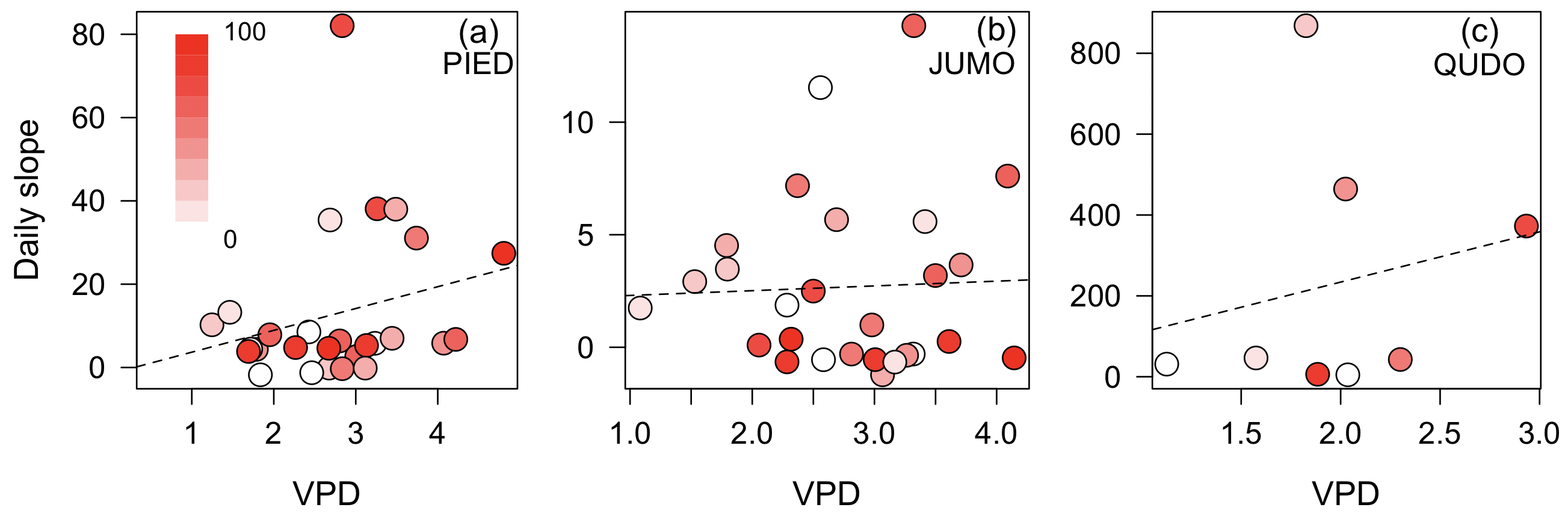The slope of the stomatal cost function is uncorrelated with vapor pressure deficit (VPD; kPa) across the tree species Pinus edulis (a), Juniperus monosperma (b), and Quercus douglasii (c). Each point is a day and the color of points is the predawn leaf (e.g. soil) water potential percentile (color bar) for that day and redder colors indicate more negative water potentials. Lines are the OLS best fit and are not statistically significant.

Figure 2: Stomatal cost function slope compared to predawn water potential.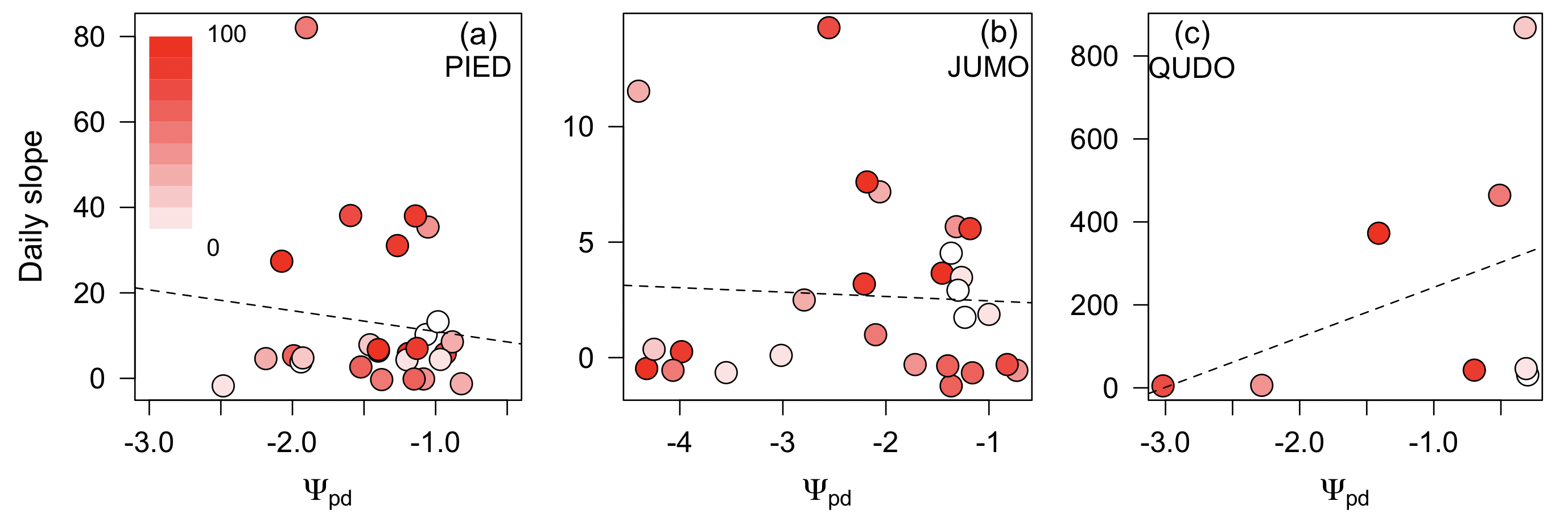The slope of the stomatal cost function is uncorrelated with predawn leaf water potential (Ψpd; MPa ) across the tree species Pinus edulis (a), Juniperus monosperma (b), and Quercus douglasii (c). Each point is a day and the color of points is the vapor pressure deficit (color bar) for that day and redder colors indicate higher VPD values. Lines are the OLS best fit and are not statistically significant.

While the predictive ability of the slope of the cost function was fairly low overall in both species with a multitude of daily data (P. edulis and J. monosperma), lagged drought indicators improved predicting cost functions (Table 1). For P. edulis, the best fitting and most parsimonious model selected by AIC with non-lagged variables explained 17% of the variation (p=0.04) and contained the highest leaf water potential recorded during that day, the number of stomatal conductance measurements, and the date. Considering lagged variables, model selection yielded the same three current-day predictor variables and also the previous date’s lowest measured leaf water potential, and the variance explained rose to 25% (p=0.03, ΔAIC>2) (Table 1). For J. monosperma, the best current-day model explained only 12% of the variation and was marginally significant (p=0.1). This model included the variables highest leaf water potential recorded during that day and the date (Table 1). Considering lagged variables as well, the most parsimonious model included the two above variables and also the maximum VPD of the previous measurement date. The variance explained in this model rose to 23% (p=0.01; ΔAIC>2) (Table 1).

Table 1 : Model selection of predicting the shadow price of stomatal behavior.

Species

Variable group

Variable

Model R2

Model p value

P. edulis

Current

LWPhigh

0.17

0.04

N measurements

Date

Current+lag

LWPhigh

0.25

0.03

N measurements

Date

LagLWPlow

J. monosperma

Current

LWPhigh

0.12

0.1

Date

Current+lag

LWPhigh

0.23

0.01

Date

LagVPDmax

Model selection results for predicting the shadow price (parameter a in Eqn. 6) from environmental variables of current or current+lagged variables.

Examining seasonal variation in cost functions, I observed moderate seasonal differences in cost functions in the two gymnosperm species (p<0.01), but not in the three angiosperm species (Fig. 3). Uncertainty in cost functions was somewhat larger in the wetter periods than drier periods, especially in the tropical angiosperm species (Fig. 3). A sensitivity analysis with an alternate vulnerability curve for Q. douglasii (Skelton et al., 2017) showed predicted gs values that were very similar to the ones presented here (R2=0.99). One potential reason for the detection of seasonal differences in the gymnosperm species may be due to biome-level differences in climate, whereby the gymnosperm species experienced a much larger variation of water potential and much greater declines in water potential during the drier periods (Fig. 4).

Despite slight seasonal differences in the gymnosperm species, the predictive ability of a single set of CM parameters at a species level was quite similar to the model allowing for seasonal differences in CM parameters (R2species=0.48; R2seas=0.50; Fig. 5). Indeed, the predictive differences between the models was minimal; the root mean squared error difference to the models was 0.002 mol m-2 sec-1 (RMSEspecies=0.077; RMSEseas=0.075). Predictive ability was strongest in the two gymnosperm and the temperate oak species and substantially lower in the two tropical species.

Figure 3: Seasonal estimates of the stomatal cost function slope parameter.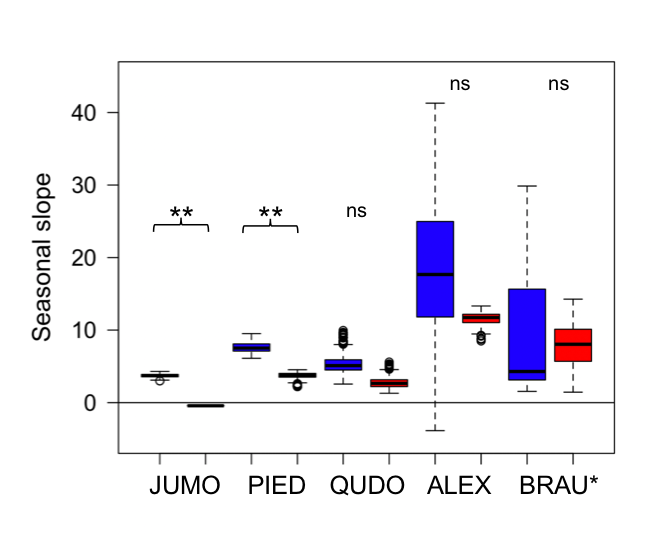Seasonal estimates of the stomatal cost function slope parameter in the wet (blue) and dry (red) periods for Juniperus monosperma (JUMO; N=576), Pinus edulis (PIED; N=511), Quercus douglasii (QUDO; N=166), Alphitonia excelsa (ALEX; N=173) and Brachychiton australis (BRAU; N=100). Black line is the median; boxes the interquartile range, and error bars show the highest and lowest value of the data excluding outliers. Stars indicate statistical significance (i.e. 95% confidence intervals do not overlap)

Figure 4: Range in predawn leaf water potential for all species.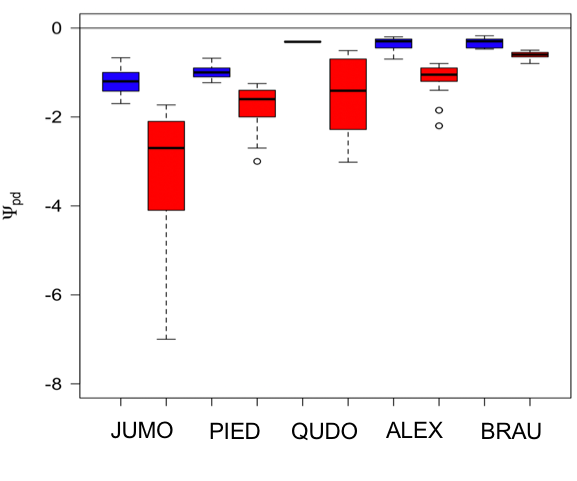Range in predawn leaf water potential for all species in the wet (blue) and dry (red) periods for Juniperus monosperma (JUMO; N=576), Pinus edulis (PIED; N=511), Quercus douglasii (QUDO; N=166), Alphitonia excelsa (ALEX; N=173) and Brachychiton australis (BRAU; N=100). Black line is the median; boxes the interquartile range, and error bars show the highest and lowest value of the data excluding outliers.

Figure 5: Observed versus predicted stomatal conductance from the CM optimization.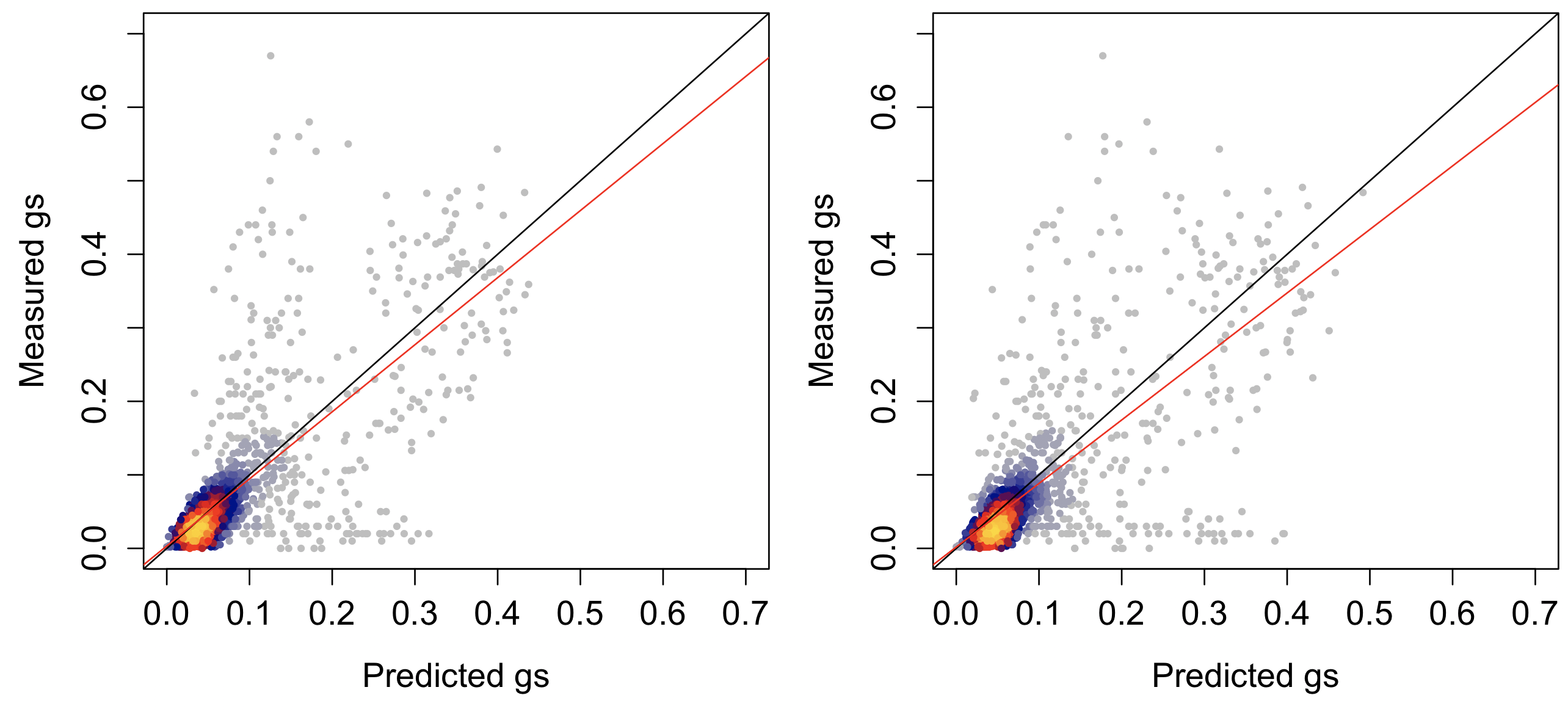Observed versus predicted stomatal conductance (gs; mol m-2 sec-1) for all five species where a separate set of parameters are used for wet and dry periods for each species (left) or a single set of parameters is used for each species (right). Colors show the density of points with gray and blue as low density and red to yellow as highest density. Red line is the ordinary least squares regression best fit and black line is the 1:1 line.

## Discussion

Predicting stomatal conductance across multiple time-scales is a central aim of many vegetation models and may be informed by optimal stomatal models. I analyzed the parameters of an optimal stomatal model at multiple time-scales and reached three central conclusions. First, the key parameter of the CM optimal stomatal model – the marginal cost of water potential – does not change predictably in response to VPD and ψs in the species analyzed here. Second, there is some evidence for the influence of lagged variables on stomatal model parameters, indicating stomata might be behaving in a somewhat Bayesian manner, though the evidence is not strong. Finally, while slight seasonal changes in parameters were detected in two of five species, a single set of species-level parameters worked nearly as well for prediction of stomatal conductance across all time-scales.

The longest and most detailed datasets of the two conifer species revealed that including previous date’s lowest leaf water potential (P. edulis) or the previous date’s maximum VPD (J. monosperma) improved prediction of the current date’s cost function slope. Previous research has documented changes in gas exchange and stomatal conductance following severe droughts, even if leaf water potential recovers (Resco et al., 2009) and, indeed, lingering effects of canopy area, hydraulic conductance, or hormonal adjustment (e.g. ABA) would likely influence stomatal conductance responses to environment (Brodribb et al., 2016; Skelton et al., 2017). Given that substantial canopy area adjustment was not observed during the measurement record and that measurements were typically ~1 month apart (Table S2) (Limousin et al., 2013), the lingering effects of previous measurements are more likely changes in tissue allocation or damage (e.g. embolism) or potentially signaling related. It is notable, however, that there was large diurnal variation in these cost function parameters at daily timescales, which is likely due to small number of data points per day.

In the CM optimization, the cost that stomata aim to avoid is formulated as an instantaneous carbon cost, but most likely includes multiple aspects of hydraulic “risk” that could play out over longer time periods (Wolf et al., 2016; Sperry et al., 2017; Anderegg et al., 2018). Sperry et al., (2017) use species’ hydraulic vulnerability curves as the risk/cost function, which drives stomata to close in order to avoid loss of hydraulic conductivity due to embolism. Much more research is needed to understand the physiology underpinning specific hydraulic risks. There are multiple potential physiological mechanisms underpinning how the hydraulic risk/cost function could increase as water potentials fall. These include direct impairment of photosynthesis , phloem loading limitations due to decreased carbon sink activity (Nikinmaa et al., 2013), leaf or xylem structural damage (including embolism), energetic costs of maintaining osmotic regulation (Hinckley et al., 1980; Bartlett et al., 2014), and shadow prices of future losses in photosynthesis due to hydraulic damage and risk of mortality (Anderegg et al., 2018).

The CM optimization shows substantial promise for inclusion into next-generation ecosystem models because plant hydraulic transport provides a mechanistic way to simulate drought stress on plants from easily measurable traits and because the CM optimization showed the highest predictive ability of stomatal conductance in previous analyses (Anderegg et al. 2018). The findings here that seasonal differences in parameters are minimal and do not greatly affect accuracy of prediction of leaf-level stomatal conductance lend further support. Intra-specific variation and plasticity in plant drought responses and hydraulic transport are likely to be important in many contexts and may not be captured in the relatively short (1-2 year) datasets included here. Plasticity or variation can be incorporated hydraulic-enabled ecosystem models through hydraulic trait variation, which will allow the CM optimization to flexibly predict stomatal conductance in a wide array of environments.

## Acknowledgements

I thank Y.S. Lin and B. Medlyn for compiling the original database from which several species’ stomatal conductance data were obtained for this study and J. Limousin, B. Choat, and D. Baldocchi for sharing data. I further thank S. MacAdam and N. Martin for their insightful reviews. W.R.L.A. acknowledges funding from the University of Utah Global Change and Sustainability Center, NSF Grant 1714972, and the USDA National Institute of Food and Agriculture, Agricultural and Food Research Initiative Competitive Programme, Ecosystem Services and Agro-ecosystem Management, grant no. 2018-67019-27850.

# Authors

#### William R. L. Anderegg

Affiliation : School of Biological Sciences, University of Utah

Country : United States

# Attachments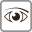Views: 1907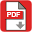PDF: 333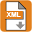XML: 112